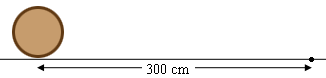SEARCH HOMEMath Central Quandaries & QueriesQuestion from andrea, a student: a wheel having a radius of 10cm rotates such that the linear speed at its rim is 30mls. what is the angular speed of the wheel in rpm?Hi Andrea,

I want to use centimeters for both distance measurements so the linear speed is 300 cms/sec. Hence in one second the wheel will travel 300 centimeters. Thus the wheel travels $60 \times 300$ centimeters in one minute.The radius of the wheel is 10 centimeters. What is its circumference? That is the distance the wheel travels in one revolution. How many revolutions does it take to travel $60 \times 300$ centimeters? How long does it take?

PennyMath Central is supported by the University of Regina and the Imperial Oil Foundation.